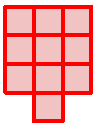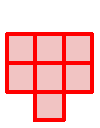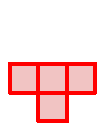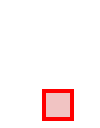### Home > CAAC > Chapter 5 > Lesson 5.1.1 > Problem5-7

5-7.

Draw Figures 1, 2, and 3 for a tile pattern that could be described by $y=−3x+10$.

Use the eTool below to create a pattern that could be described by $y=−3x+10$.
Click the link to the right to view full version. CCA 2-34 HW eTool.

Use figure zero as a starting point. The growth is $−3$, so remove three tiles for the next figure.Figure 0 Figure 1 Figure 2 Figure 3